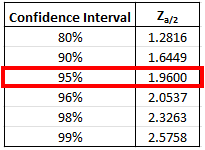## Confidence Interval

To test for yield strength, consider 40 aluminum test specimens were subjected to a tensile load test. If the standard deviation is 2.5 and the sample mean is 200 MPa, what is the 95% confidence interval for the aluminum’s yield strength?

Hint
The population’s standard deviation, $$\sigma$$ , is known, and the sample size is greater than 30. Thus, we need to use the z distribution. We would only use the t distribution if $$\sigma$$ is unknown and if the sample size is less than 30.
Hint 2
The Confidence Interval for the Mean µ of a Normal Distribution when a standard deviation $$\sigma$$ is known:
$$\bar{X}-Z_{a/2}\frac{\sigma}{\sqrt{n}}\leq \mu \leq \bar{X}+Z_{a/2}\frac{\sigma}{\sqrt{n}}$$$where $$\bar{X}$$ is the sample mean, $$\sigma$$ is the standard deviation, $$n$$ is the sample size, and $$Z_a$$ corresponds to the appropriate probability under the normal probability curve for a given $$Z_{var}$$ (standard normal Z score). The population’s standard deviation, $$\sigma$$ , is known, and the sample size is greater than 30. Thus, we need to use the z distribution. We would only use the t distribution if $$\sigma$$ is unknown and if the sample size is less than 30. The Confidence Interval for the Mean µ of a Normal Distribution when a standard deviation $$\sigma$$ is known: $$\bar{X}-Z_{a/2}\frac{\sigma}{\sqrt{n}}\leq \mu \leq \bar{X}+Z_{a/2}\frac{\sigma}{\sqrt{n}}$$$
where $$\bar{X}$$ is the sample mean, $$\sigma$$ is the standard deviation, $$n$$ is the sample size, and $$Z_a$$ corresponds to the appropriate probability under the normal probability curve for a given $$Z_{var}$$ (standard normal Z score). $$Z_{a/2}$$ is usually derived from confidence interval table (like in the FE exam handbook):Therefore, the 95% confidence interval is:
$$200-1.96\frac{2.5}{\sqrt{40}}\leq \mu \leq 200+1.96\frac{2.5}{\sqrt{40}}$$$$$200-1.96(0.395)\leq \mu \leq 200+1.96(0.395)$$$
$$200-0.775\leq \mu \leq 200+0.775$$$$$199.22\leq \mu \leq 200.78$$$
Thus, the answer is (199.22, 200.78)
(199.22, 200.78)